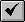English

# How To: Add direction arrows to arcs using Avenue

## Summary

```Note:
The information in this article applies to ArcView GIS versions 3.x only. The instructions described apply to the Avenue programming language, which is specific to the ArcView GIS application.```

The script in this article shows how to add directional arrow symbols to selected features of a polyline theme.

## Procedure

This example requires a view, selected features of a polyline theme, Fnode and Tnode fields in the attribute table of the Polyline theme.

1. Open a new script window.
1. Activate the Project window.
2. Click the Scripts icon.
3. Click New.
2. Copy the following code into the new window:
```'-- Name: AVArrows.ave
'
'-- This script creates a line theme of arrows showing arc
'-- direction of another line theme.
'-- This script facilitates editing of shapefiles and
'-- attributes when line direction is important, such as
'-- editing the address ranges of a street file for geocoding.
'
'-- Before running it, make a polyline theme active,
'-- select some of the lines (with a selection tool).
'
'-- Self: N/A
'-- Returns: N/A
'-- Topics: shape, direction, arcarrows
'-- Search Keys: shape, direction, arcarrows
'-- Requires:  companion script MakeArrow.ave

'-- Create the output object
out_name = FileDialog.Put( "*.shp".asfilename, "*.shp", "Output Shapefile")

if (out_name = nil) then
exit
end

arrowIDfld = Field.Make("Arrow_ID",#FIELD_SHORT,6,0)
fields  = list.Make
tblrecno=0

'-- Create the new Ftab
tbl = Ftab.MakeNew( out_name, Polyline )

v = av.GetActiveDoc
t = v.GetThemes.Get(0)
d = v.GetDisplay

tFTab = t.GetFTab

'-- Find a good length for the arrow lines
l = (d.ReturnExtent.GetWidth)/80

tShape = tFTab.FindField("Shape")

tGraphicSet = t.GetGraphics
vGraphics   = v.GetGraphics

theNumSelected = tFTab.GetSelection.Count
theCounter = 0
av.ShowMsg("Working...")

for each r in tFTab.GetSelection
theCounter = theCounter + 1
av.SetStatus((theCounter/theNumSelected) * 100)

pntListList = (tFTab.ReturnValue(tShape,r)).AsList

for each pntList in pntListList

numPoints = pntList.Count

for each i in 1..(numPoints-1)

Fpnt = pntList.Get((i-1))
Tpnt = pntList.Get(i)

tbl.SetValue(tbl.FindField("Shape"), outputRecNum, arrowShape)
tbl.SetValue(arrowIDfld, outputRecNum, outputRecNum)

end
end
end

av.ClearStatus
av.ClearMsg
theFTheme = FTheme.Make(tbl)
theFTheme.SetVisible(true)
MsgBox.Info("Created Arrow Theme","Done")
```
1. Select Properties from the Script menu.
2. Type AVArrows.ave for the name of the script.
3. Click OK.
4. Select Compile from the Script menu, or click the Compile button.5. Open another new script window.
6. Copy the following code into the new window:
```'-- Name: MakeArrow.ave
'
'-- This script builds an arrow polyline (2 points) for displaying
'-- the orientation of a line. It is required by AVArrows.ave.
'
'-- Self: {FromPoint,ToPoint,Angle,Length}
'-- Returns: aPolyline
'-- Topics: shape, direction, arcarrows
'-- Search Keys: shape, direction, arcarrows
'-- Requires: N/A

fp     = self.Get(0)    '-- from point
tp     = self.Get(1)    '-- to point
theta  = self.Get(2)    '-- angle
length = self.Get(3)    '-- length

x1 = tp.GetX
y1 = tp.GetY
x2 = fp.GetX
y2 = fp.GetY

xshift = length * (theta.cos)
yshift = length * (theta.sin)
totLength = (((y1-y2)^2)+((x1-x2)^2)).Sqrt

'-- Point along the line
p1 = ((1.0 - (xshift/totLength)) * x1) + ((xshift/totLength) *x2)
p2 = ((1.0 - (xshift/totLength)) * y1) + ((xshift/totLength) *y2)

pi = Number.GetPI

if (x2<>x1) then
slope = (y2-y1)/(x2-x1)
slant = (slope/(((slope^2)+1).Sqrt)).ASin
else
slant = pi/2.0
end

a1 = p1 - (yshift * (slant.sin))
a2 = p2 + (yshift * (slant.cos))

b1 = p1 + (yshift * (slant.sin))
b2 = p2 - (yshift * (slant.cos))

lpnt = Point.Make(a1,a2)
rpnt = Point.Make(b1,b2)

aPolyLine = PolyLine.Make({{lpnt,tp},{tp,rpnt}})

return(aPolyline)
```
1. Select Properties from the Script menu.
2. Type MakeArrow.ave as the name of the script.
3. Click OK.
4. Compile the script.
5. Attach the AVArrows.ave script to a button on the View GUI.
1. Compile the script.
2. Switch to the Project window.
3. Select Customize from the Project menu.
4. On the Customize dialog box, click the Type drop-down arrow and click View.
5. Select Buttons under Category.
6. Click the New button.
7. Double-click the Click property in the Customize dialog box.
8. Type the name of the script in the Script Manager and click Select.
9. Close the Customize dialog box.